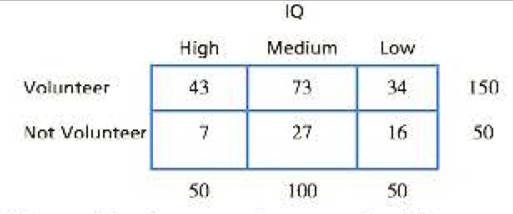Chapter 15, Problem 21PEssentials of Statistics for the B...

8th Edition
Frederick J Gravetter + 1 other
ISBN: 9781133956570

Solutions

Chapter
SectionEssentials of Statistics for the B...

8th Edition
Frederick J Gravetter + 1 other
ISBN: 9781133956570
Textbook Problem

Research indicates that people who volunteer to participate in research studies tend to have higher intelligence than nonvolunteers. To test this phenomenon, a researcher obtains a sample of 200 high-school students. The students are given a description of a psychological research study and asked whether they would volunteer to participate. The researcher also obtains an IQ score for each student and classifies the students into high, medium, and low IQ groups. Do the following data indicate a significant relationship between IQ and volunteering? Test at the .05 level of significance.To determine

To check: Whether there is significant relationship between the IQ and volunteering for the given question.

Explanation

Given info:

A sample of 200 students were involved in a study based on “the IQ score of a student and their willingness to volunteer”. Use α=0.05 to test the claim.

Calculations:

Step 1: Null Hypothesis and Alternate Hypothesis are:

H0: No significant relationship between the IQ and volunteering.

H1: Significant relationship between the IQ and volunteering.

Step 2: For the given sample, degrees of freedom equals:

df=(R1)(C1)  where R equals number of rows and C equals columns=(21)(31)=2

With α=0.05 and df=2, the critical value (CV)  is obtained from the χ2table as

χ2=5.991

Step 3: χ2statistics is calculated as:

χ2=(fofe)2fe

The formula to calculate expected frequency is:

fe=fcfrnwhere fr is row frequency and fc is column frequency

Substitute n=200 in the above formula and compute respective values of expected frequencies:

For the category “volunteer”, the expected frequencies are:

fe,high=50×150200fe,medium=100×150200fe,low=50<

Still sussing out bartleby?

Check out a sample textbook solution.

See a sample solution

The Solution to Your Study Problems

Bartleby provides explanations to thousands of textbook problems written by our experts, many with advanced degrees!

Get Started

Evaluate the integral. 39. cscxdx

Single Variable Calculus: Early Transcendentals

The general solution to (for x, y > 0) is: a) y = ln x + C b) c) y = ln(ln x + C) d)

Study Guide for Stewart's Single Variable Calculus: Early Transcendentals, 8th

True or False: is conservative.

Study Guide for Stewart's Multivariable Calculus, 8th

Define content analysis and archival research.

Research Methods for the Behavioral Sciences (MindTap Course List)